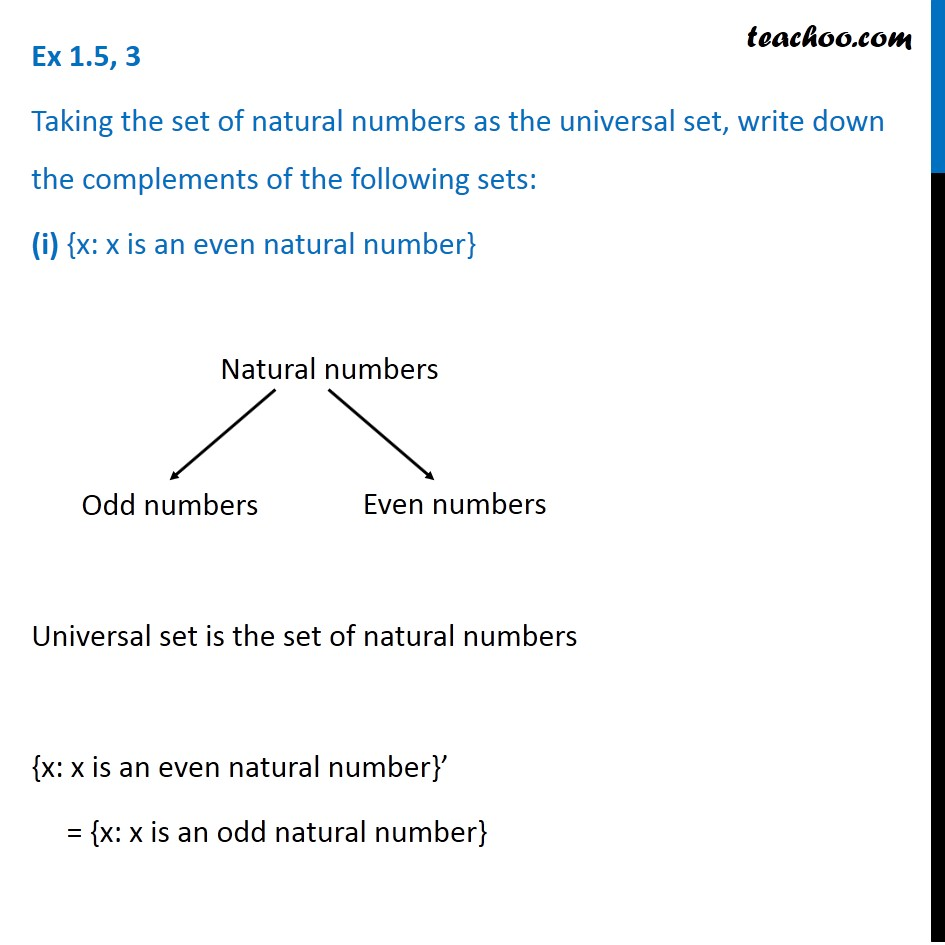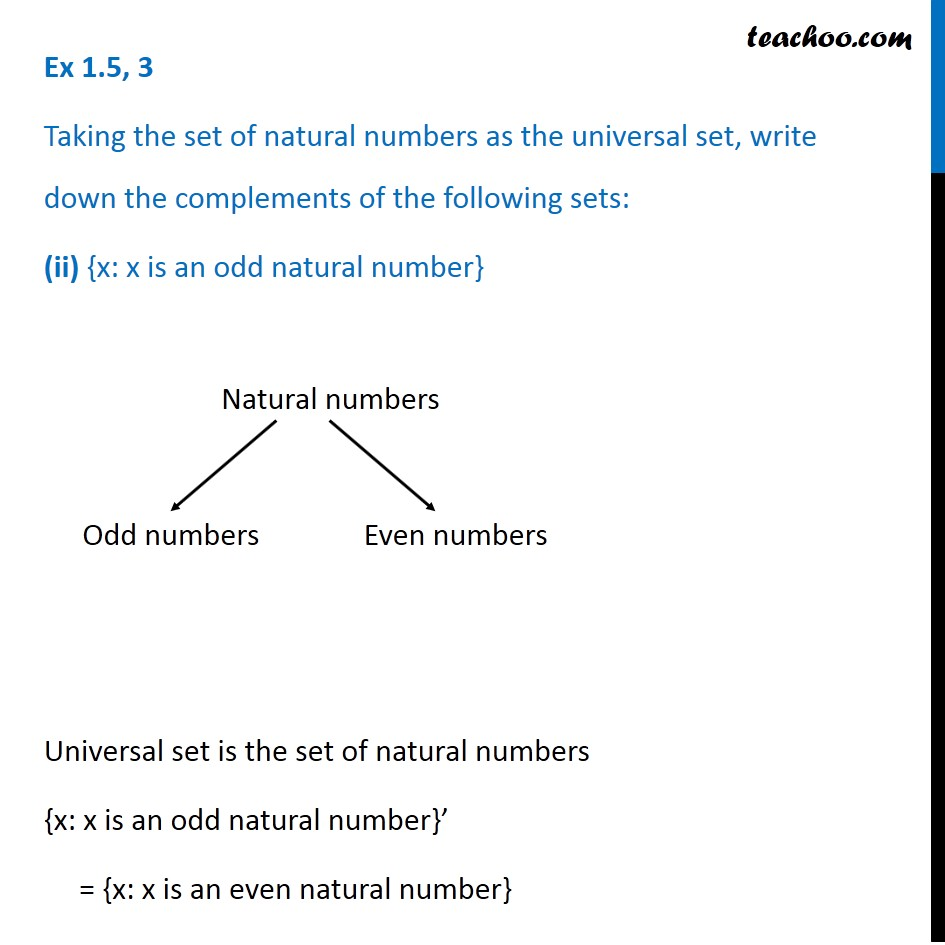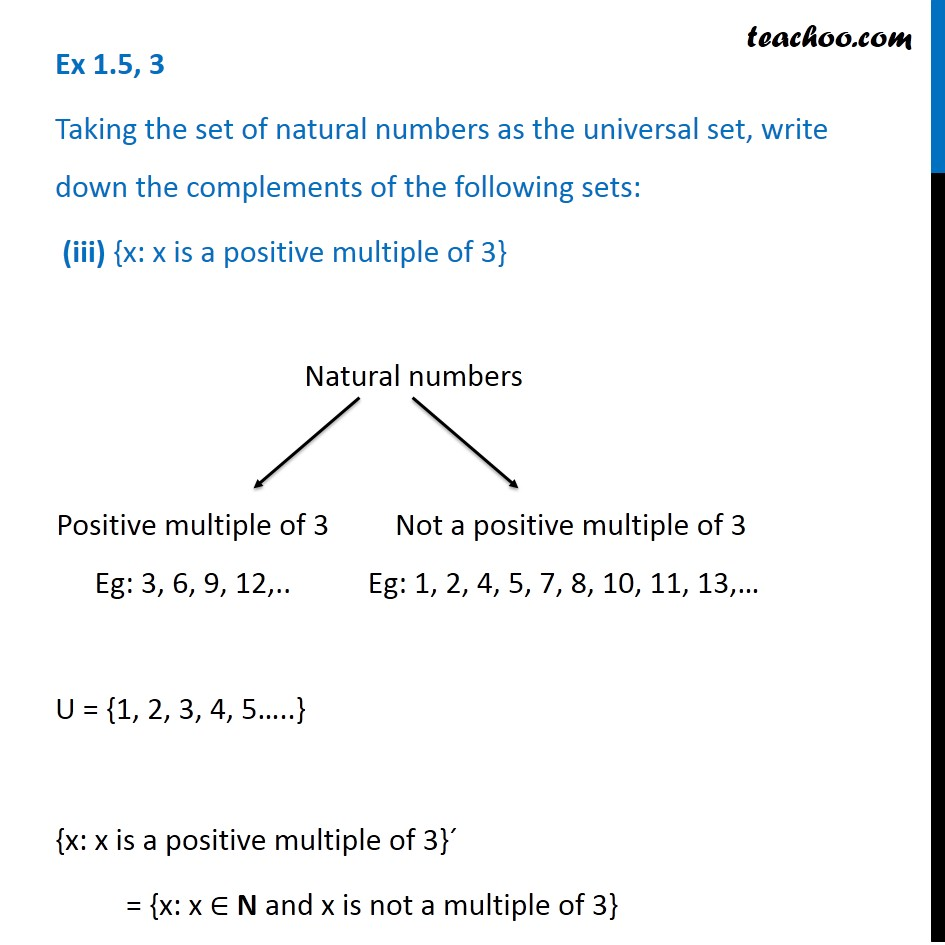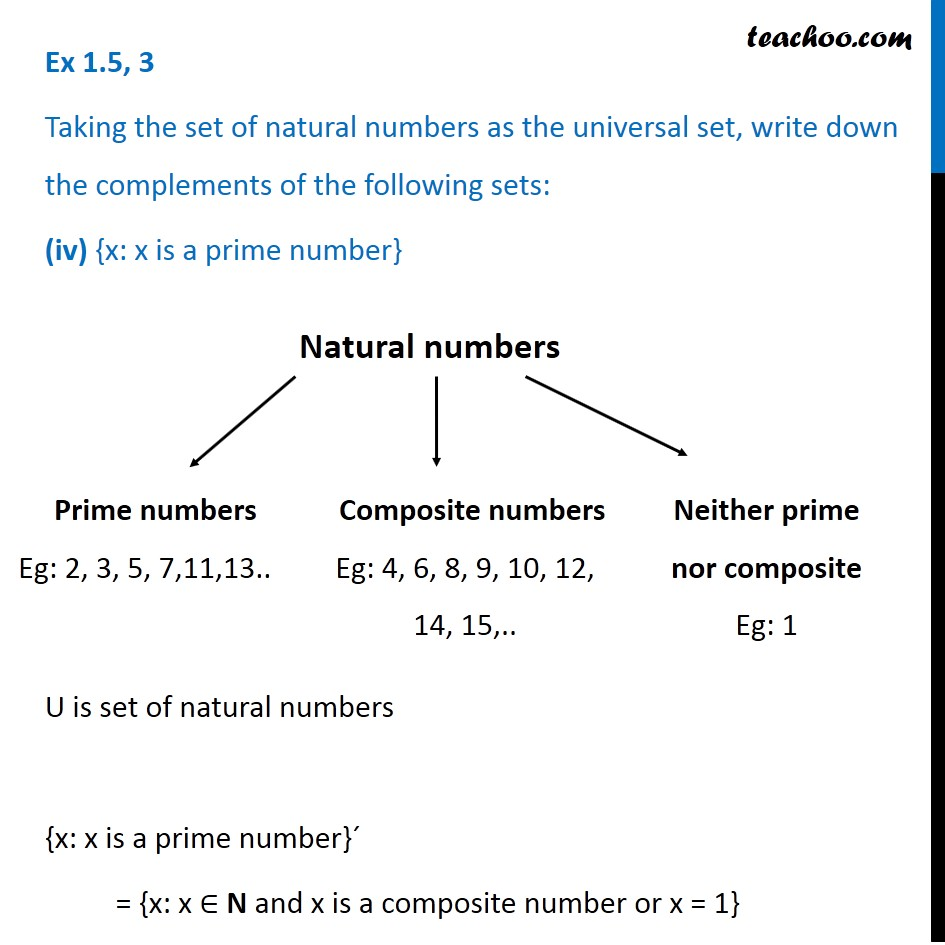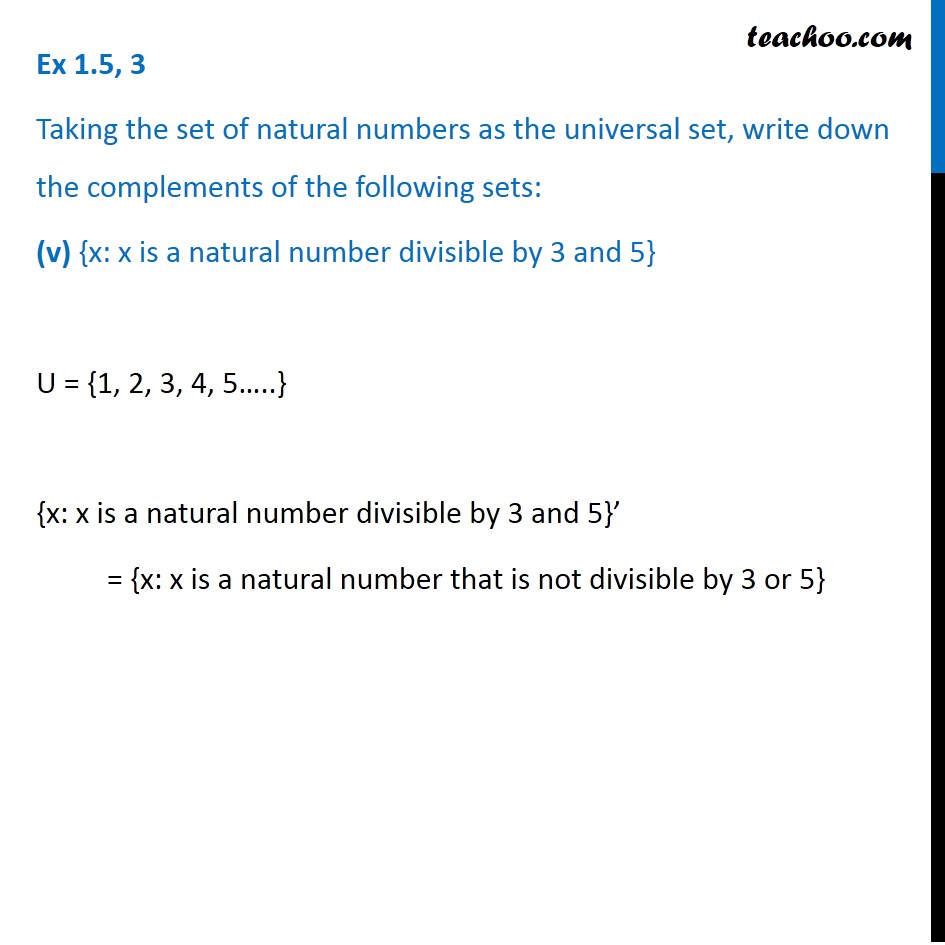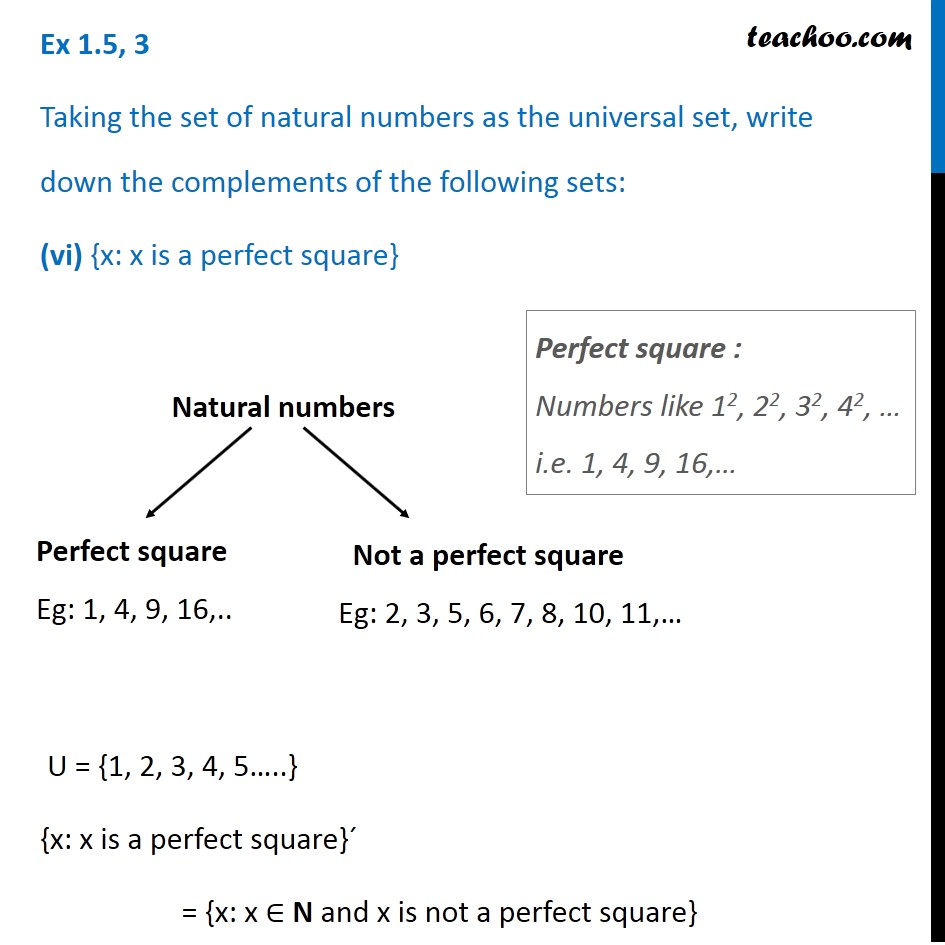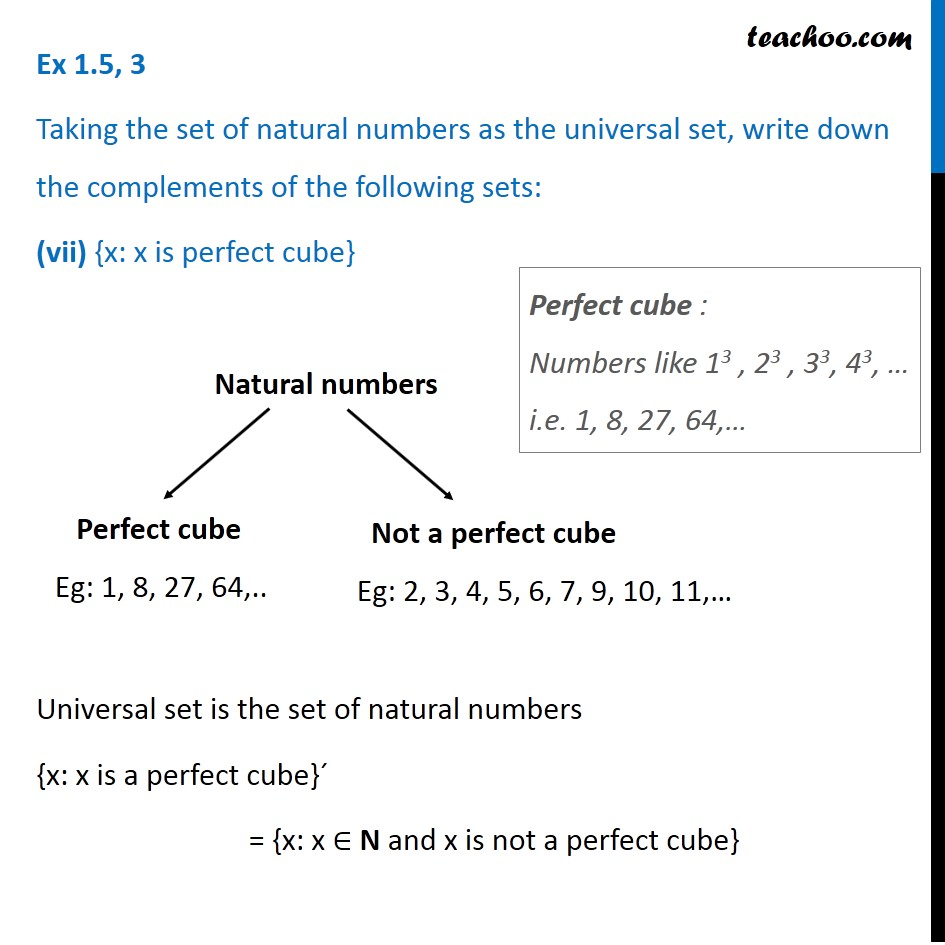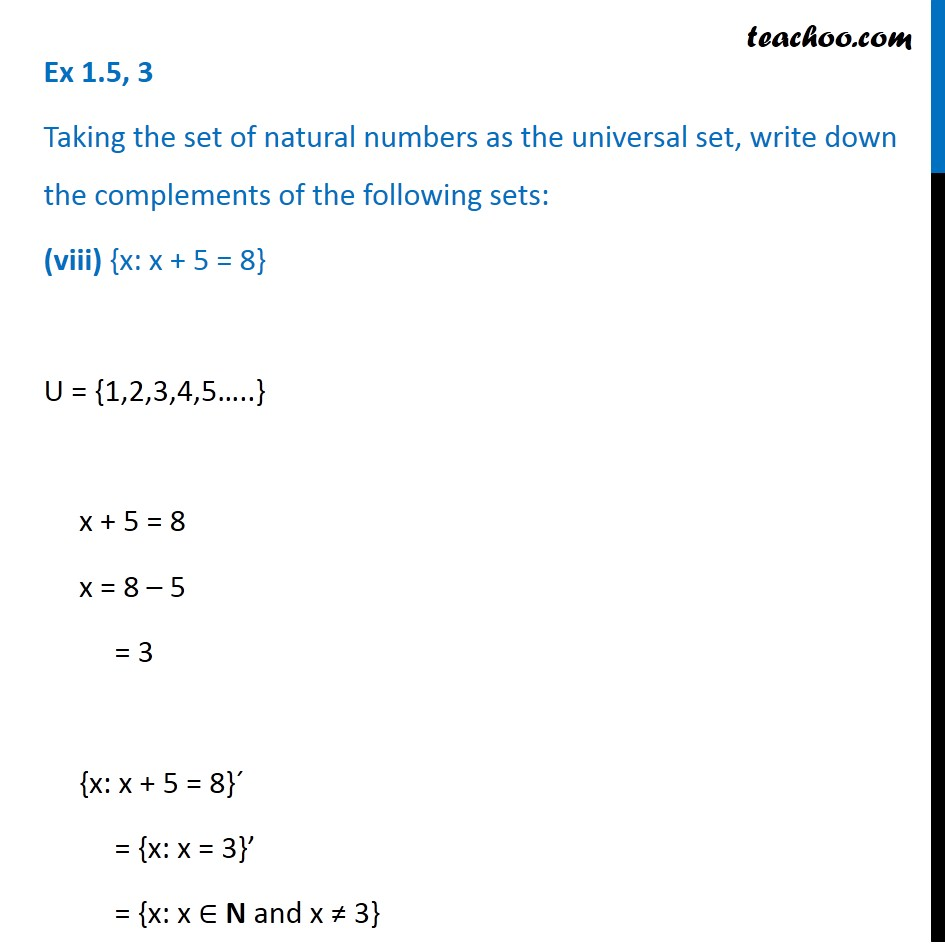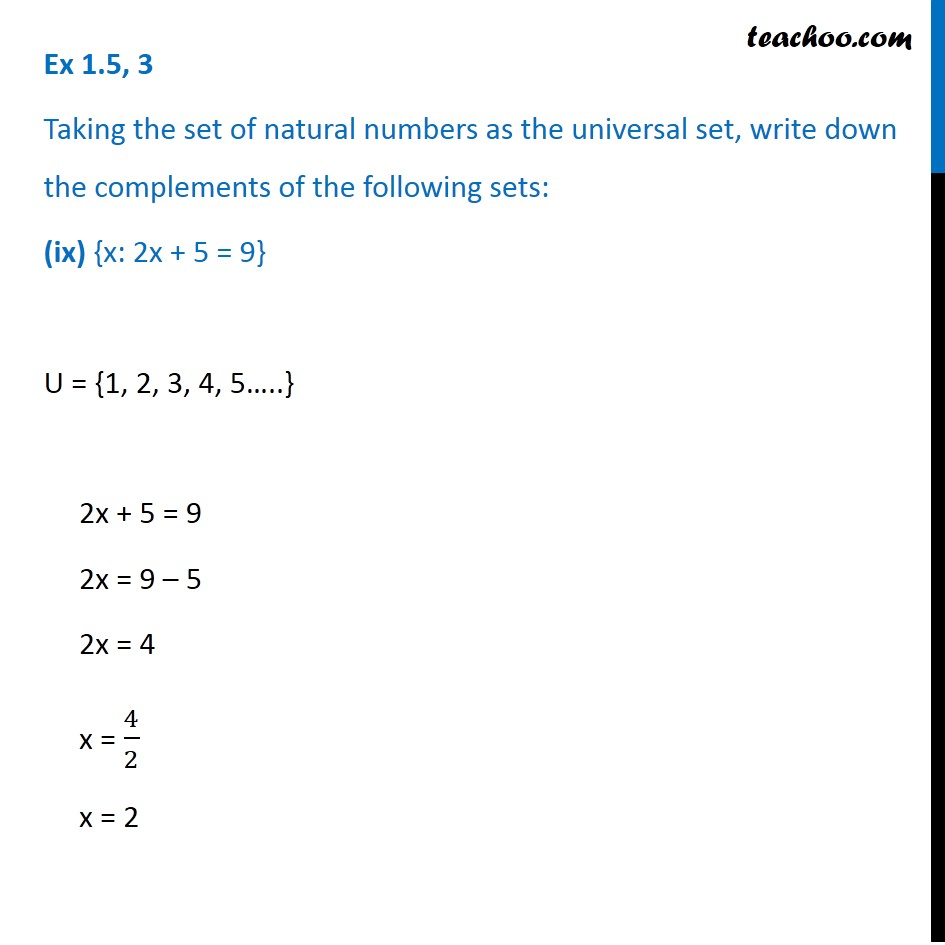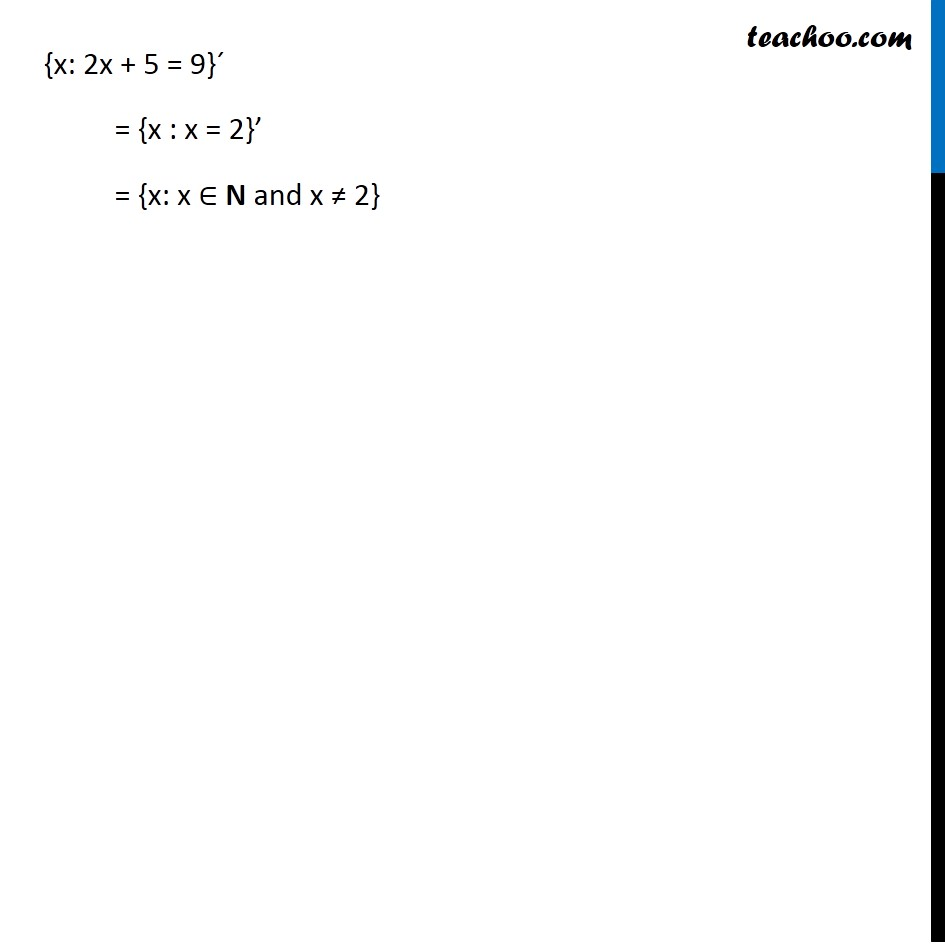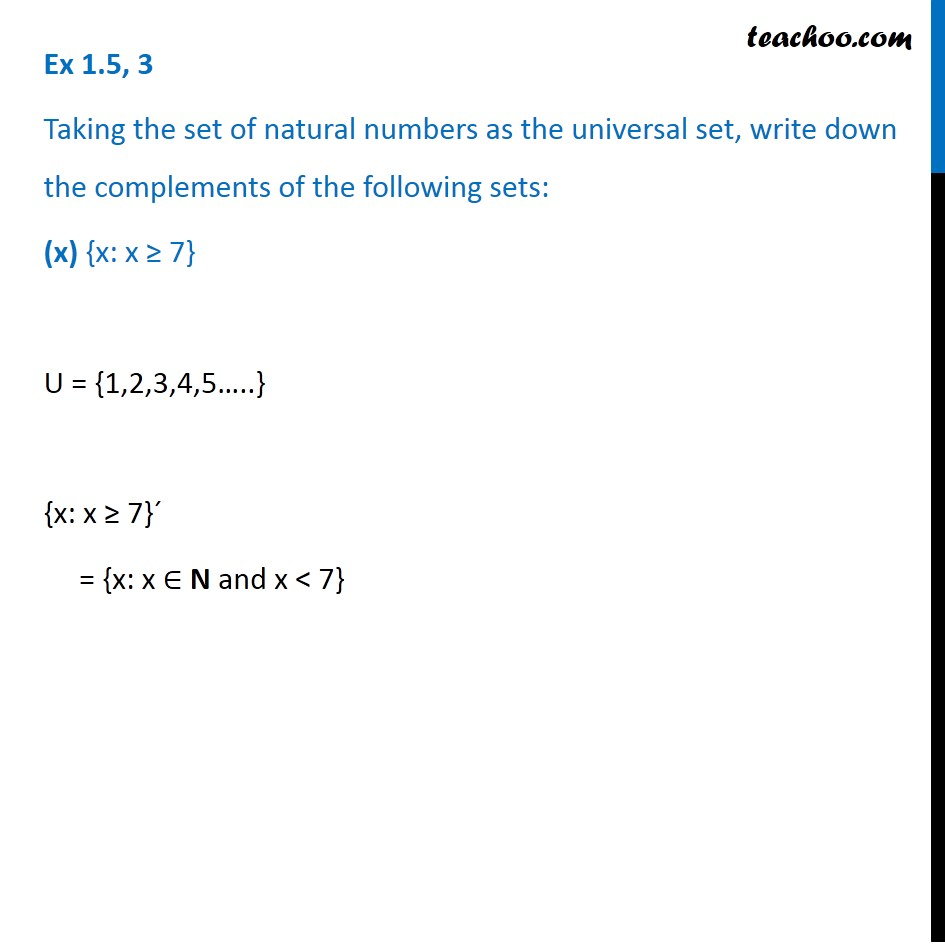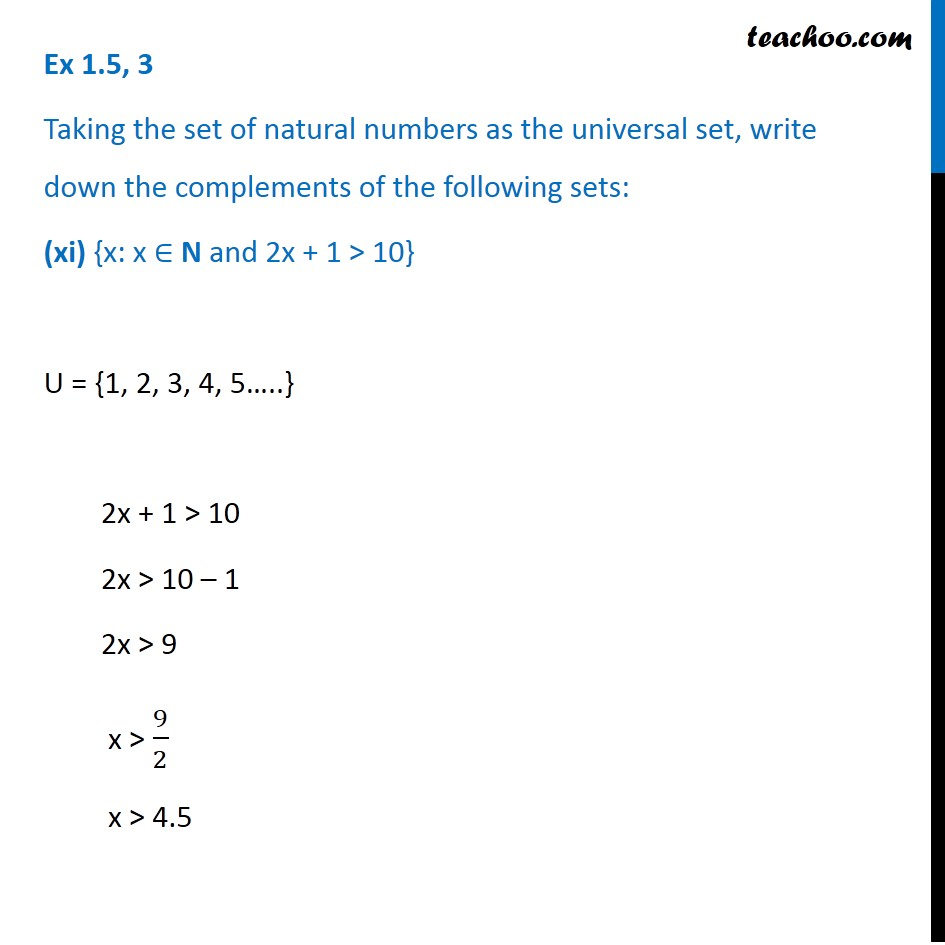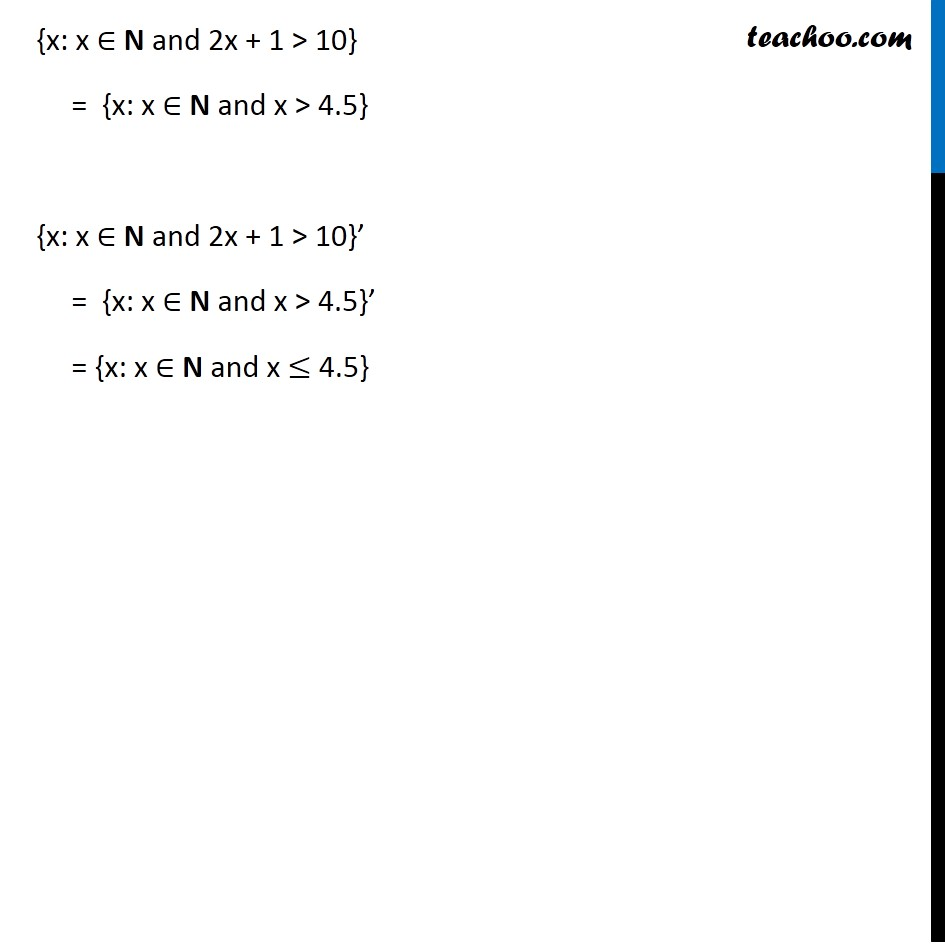1. Chapter 1 Class 11 Sets
2. Serial order wise
3. Ex 1.5

Transcript

Ex 1.5, 3 Taking the set of natural numbers as the universal set, write down the complements of the following sets: (i) {x: x is an even natural number} Universal set is the set of natural numbers {x: x is an even natural number}’ = {x: x is an odd natural number} Ex 1.5, 3 Taking the set of natural numbers as the universal set, write down the complements of the following sets: (ii) {x: x is an odd natural number} Universal set is the set of natural numbers {x: x is an odd natural number}’ = {x: x is an even natural number} Ex 1.5, 3 Taking the set of natural numbers as the universal set, write down the complements of the following sets: (iii) {x: x is a positive multiple of 3} U = {1, 2, 3, 4, 5…..} {x: x is a positive multiple of 3}´ = {x: x ∈ N and x is not a multiple of 3} Positive multiple of 3 Eg: 3, 6, 9, 12,.. Not a positive multiple of 3 Eg: 1, 2, 4, 5, 7, 8, 10, 11, 13,… Ex 1.5, 3 Taking the set of natural numbers as the universal set, write down the complements of the following sets: (iv) {x: x is a prime number} U is set of natural numbers {x: x is a prime number}´ = {x: x ∈ N and x is a composite number or x = 1} Ex 1.5, 3 Taking the set of natural numbers as the universal set, write down the complements of the following sets: (iv) {x: x is a prime number} U is set of natural numbers {x: x is a prime number}´ = {x: x ∈ N and x is a composite number or x = 1} Composite numbers Eg: 4, 6, 8, 9, 10, 12, 14, 15,.. Neither prime nor composite Eg: 1 Ex 1.5, 3 Taking the set of natural numbers as the universal set, write down the complements of the following sets: (v) {x: x is a natural number divisible by 3 and 5} U = {1, 2, 3, 4, 5…..} {x: x is a natural number divisible by 3 and 5}’ = {x: x is a natural number that is not divisible by 3 or 5} Ex 1.5, 3 Taking the set of natural numbers as the universal set, write down the complements of the following sets: (vi) {x: x is a perfect square} U = {1, 2, 3, 4, 5…..} {x: x is a perfect square}´ = {x: x ∈ N and x is not a perfect square} Perfect square Eg: 1, 4, 9, 16,.. Not a perfect square Eg: 2, 3, 5, 6, 7, 8, 10, 11,… Perfect square : Numbers like 12, 22, 32, 42, … i.e. 1, 4, 9, 16,… Ex 1.5, 3 Taking the set of natural numbers as the universal set, write down the complements of the following sets: (vii) {x: x is perfect cube} Universal set is the set of natural numbers {x: x is a perfect cube}´ = {x: x ∈ N and x is not a perfect cube} Perfect cube Eg: 1, 8, 27, 64,.. Not a perfect cube Eg: 2, 3, 4, 5, 6, 7, 9, 10, 11,… Perfect cube : Numbers like 13 , 23 , 33, 43, … i.e. 1, 8, 27, 64,… Ex 1.5, 3 Taking the set of natural numbers as the universal set, write down the complements of the following sets: (viii) {x: x + 5 = 8} U = {1,2,3,4,5…..} x + 5 = 8 x = 8 – 5 = 3 {x: x + 5 = 8}´ = {x: x = 3}’ = {x: x ∈ N and x ≠ 3} Ex 1.5, 3 Taking the set of natural numbers as the universal set, write down the complements of the following sets: (ix) {x: 2x + 5 = 9} U = {1, 2, 3, 4, 5…..} 2x + 5 = 9 2x = 9 – 5 2x = 4 x = 4/2 x = 2 {x: 2x + 5 = 9}´ = {x : x = 2}’ = {x: x ∈ N and x ≠ 2} Ex 1.5, 3 Taking the set of natural numbers as the universal set, write down the complements of the following sets: (x) {x: x ≥ 7} U = {1,2,3,4,5…..} {x: x ≥ 7}´ = {x: x ∈ N and x < 7} Ex 1.5, 3 Taking the set of natural numbers as the universal set, write down the complements of the following sets: (xi) {x: x ∈ N and 2x + 1 > 10} U = {1, 2, 3, 4, 5…..} 2x + 1 > 10 2x > 10 – 1 2x > 9 x > 9/2 x > 4.5 {x: x ∈ N and 2x + 1 > 10} = {x: x ∈ N and x > 4.5} {x: x ∈ N and 2x + 1 > 10}’ = {x: x ∈ N and x > 4.5}’ = {x: x ∈ N and x ≤ 4.5}

Ex 1.5

Chapter 1 Class 11 Sets
Serial order wise

About the AuthorDavneet Singh
Davneet Singh is a graduate from Indian Institute of Technology, Kanpur. He has been teaching from the past 10 years. He provides courses for Maths and Science at Teachoo.